# How to Display/Show Formulas in Excel

If you are working on an Excel worksheet with a lot of formulas in it, it may become difficult to follow and keep track of all your formulas. Excel provides a simple way of displaying formulas in the cells instead of the result. This Excel Trick will help you to Display/Show Formulas in Excel without any issues. Similarly, for more such tips & tricks you can follow our Excel Ninja Training and become an expert in Excel.

Imagine that when you add formulas to your Excel spreadsheet, they are hidden when printing and viewing the sheet. If you’d rather show the formulas on your sheet instead of the formula’s results, you can do it using three ways which will be discussed in this tutorial.

Excel Vlookup formula – Guidebook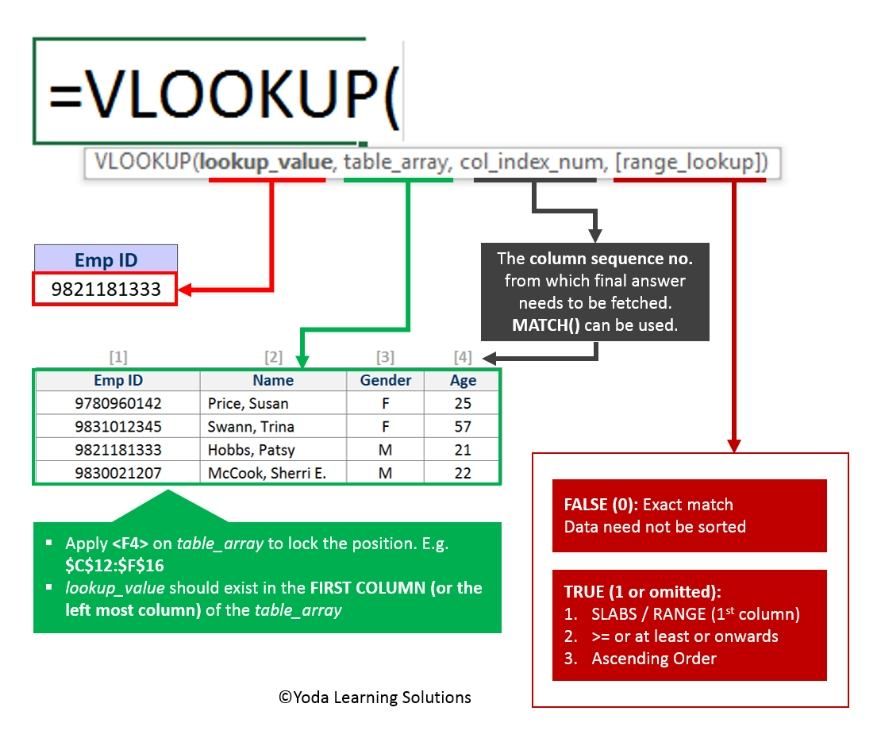If Yes, you will enjoy this guidebook on ‘Excel Vlookup Formulas’ – VLOOKUP, HLOOKUP, MATCH & INDEX.

## How to Display/Show Formulas in Excel?

So, Let’s Show Formulas in Excel Instead of the Values with those three ways!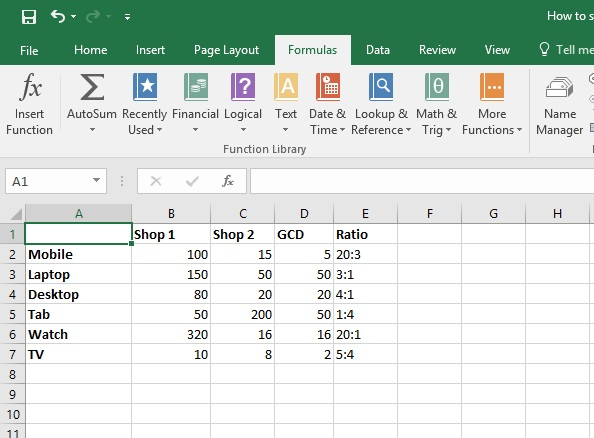### Way 1: Shortcut Command!

Step 1: Say we have a table the same as the above picture. This table is showing the number of sales of two electronics shops and column E is showing the ratio between these two shop’s sales for each product. Cells of Column D and E have a formula which is calculating the ratio. Now we want to display the formulas of these cells so that we can print it.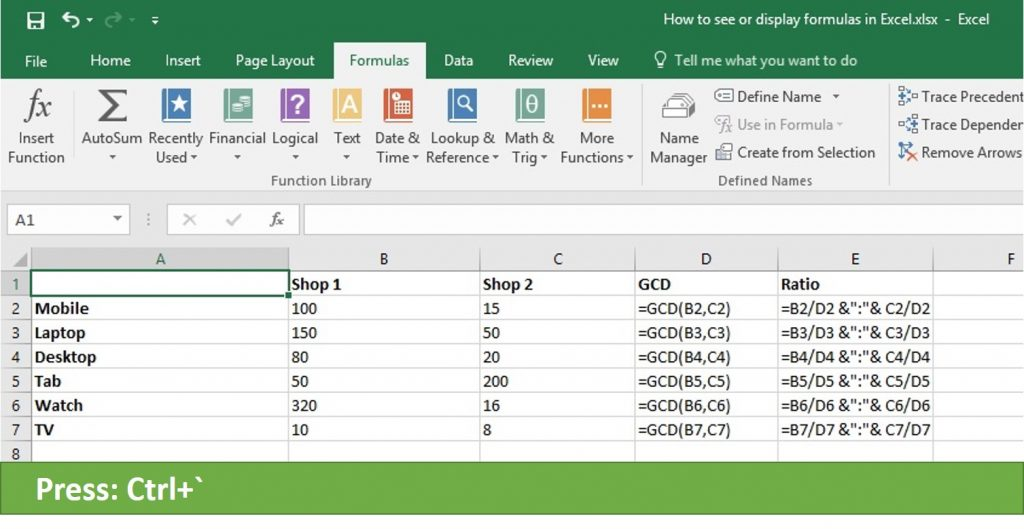Here is the shortcut way:

Just press Ctrl+`from your keyboard and see the Magic! All formulas of column D and E are showing in our excel sheet as like the above picture.

“ ` ” this symbol you can find on the left side of key 1 on your keyboard.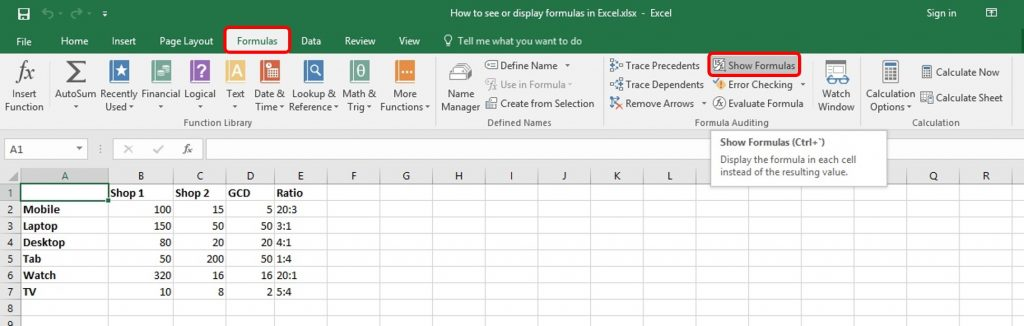### Way 2: From Formula tab!:

Now let see another way to show formula from the Menu bar.

• Step 1: Click on the ‘Formulas’ tab in the ribbon.
• Step 2: In the Formula Audition Group Click ‘Show Formulas’
• Step 3: Result: You can sell all formulas inside cells.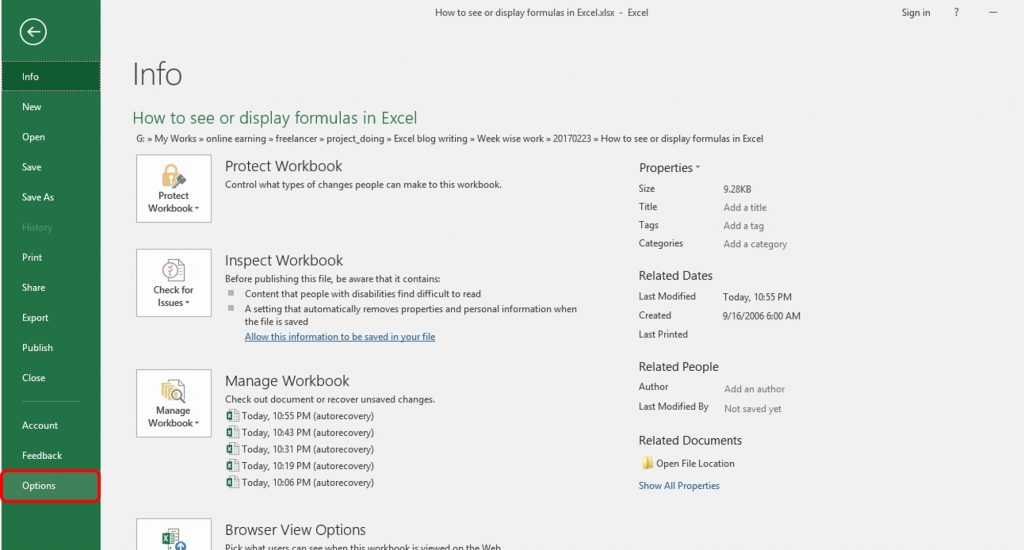Excel Vlookup formula – GuidebookIf Yes, you will enjoy this guidebook on ‘Excel Vlookup Formulas’ – VLOOKUP, HLOOKUP, MATCH & INDEX.

### Way 3: from File tab!

Step 1: Click on the File tab to move into the Backstage View. Once in Backstage View, from the drop-down menu select the category Options from the left as shown above picture.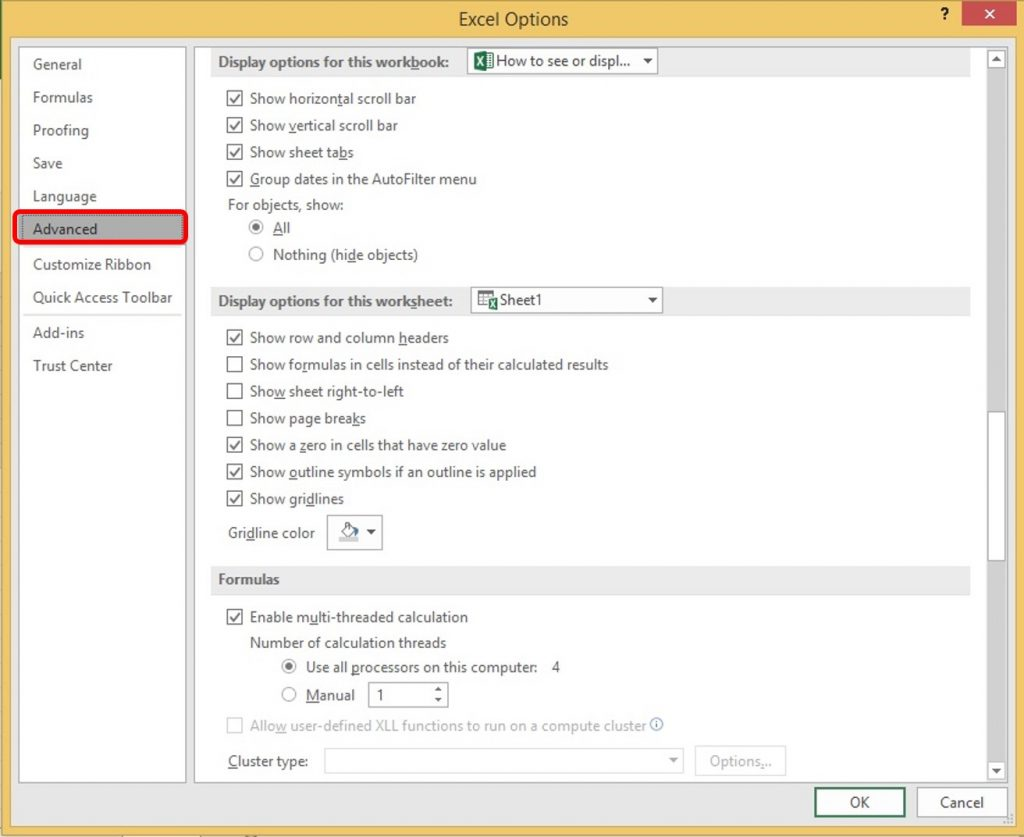Step 2: Now the Excel Options dialog box appears, we select the Advanced from the left side as like above picture.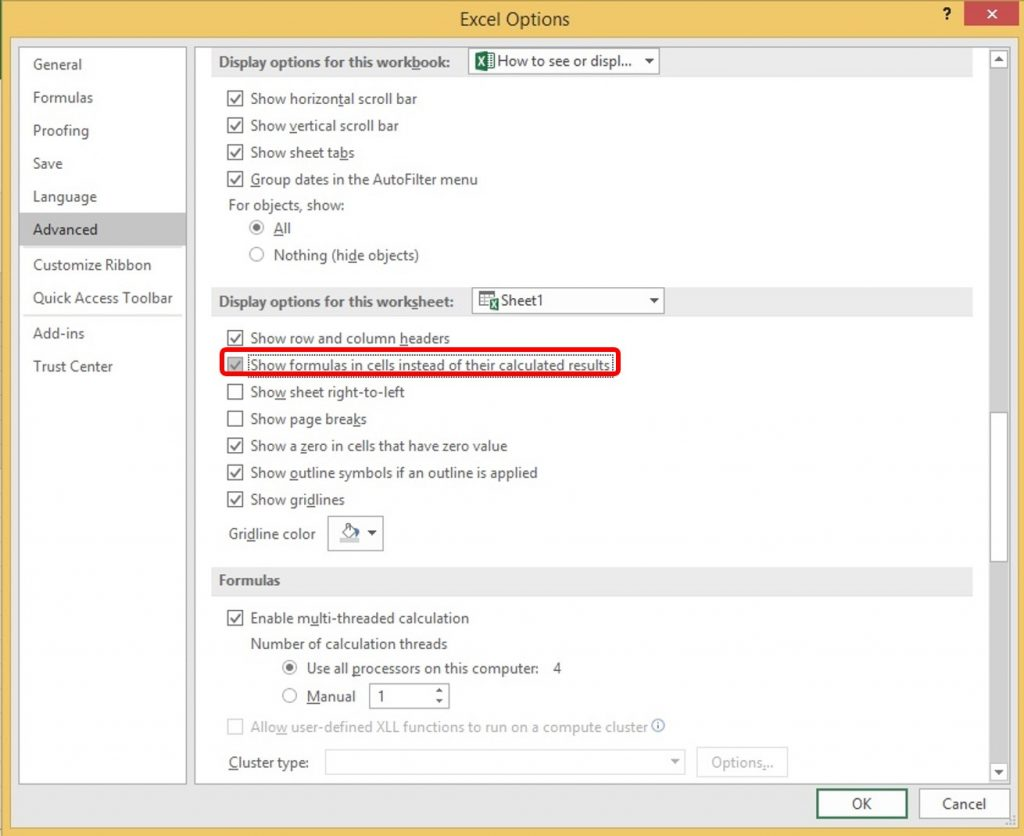Step 3: Once the Advanced category is selected, scroll down in order to locate the area named Display Options For This Worksheet. Check Show Formulas In Cells Instead Of Their Calculated Results. Once activated, press the OK button on the Excel Options dialog box in order to return to our Worksheet.

15 Excel Data Cleaning Tricks Guidebook

Bored of downloading text heavy / copy-pasted eBooks? If Yes, you will enjoy this guidebook (43 pages)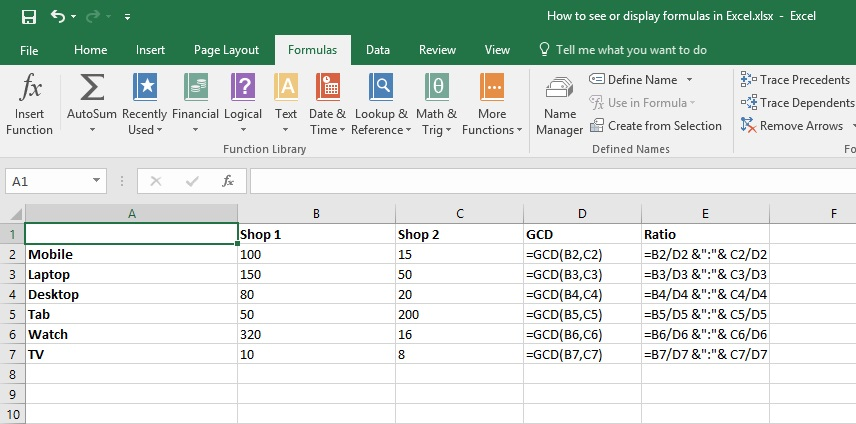Now all formulas are showing in all cells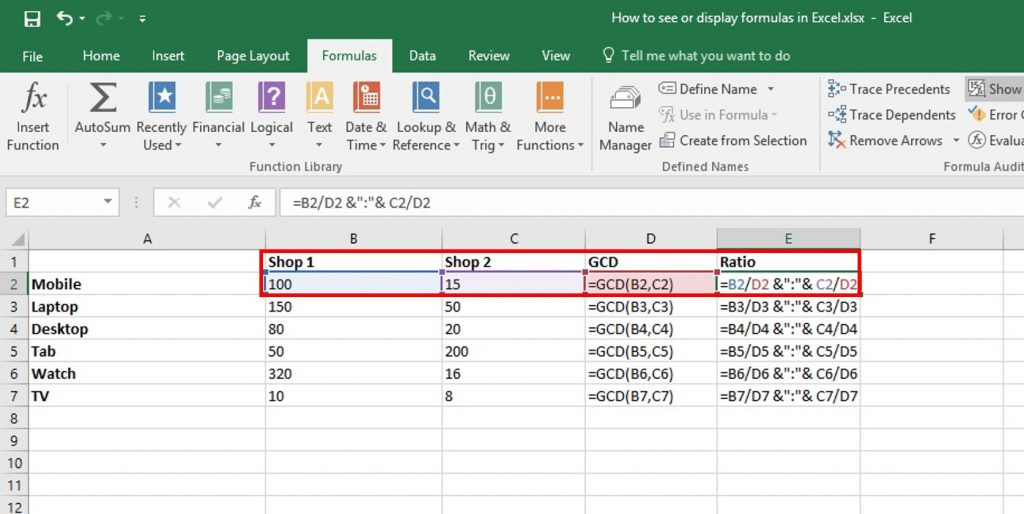Tips 1: After checking on Show Formulas In Cells Instead Of Their Calculated Results we can see that Show Formulas is also activated from the Formula tab. If we click on it again, then formulas will be invisible.

Tips 2: When you activate Show Formulas and formulas are visible and If you need to see those cells which are included inside the formula of a particular cell, You can see this by a single click in that cell that contains a formula. Say, in our this example we want to see those cells which are included in the formula of cell E2. So, we just need to single click on E2, then beside E2, those included cells are also selected with different colors. Here B2, C2, and D2 are selected.

15 Pivot Tables Tricks for Pros

Most Popular Tricks are #3, #7 & #12